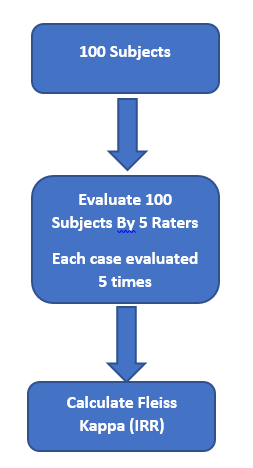# Study Design for Subjective Multinomial Outcome

for a psychological assessment 100 cases were reviewed by 5 raters to set an ordinal outcome (low - standard - high - very high). Is it enough to calculate inter-rater reliability (IRR) for only 25 randomly selected cases (sample size calculated for ICC using PASS) and then the remaining cases to be evaluated by only one rater ? Or is it a MUST that all cases to be evaluated by the 5 raters?
I mean, should the study design be like thisOr like this

Also to choose a “final” outcome from many raters, should I take the majority or calculate an average score?

Kendall tau concordance for inter-rater reliability is also an option for ordinal outcome, since Fleiss Kappa doesn’t take into account the ordinal nature of the outcome.

A consultation with a respected colleague resulted in the following:

“If the 95% CI lies within the acceptable agreement, this would justify dependence on only one rater.”

Why are you using 0.6 as a threshold in design 2? What is the advantage of randomly evaluating 25 and having 75 rated by an individual, aside from economic considerations?

If I’m thinking about this correctly, design 2 \lt design 1 if the objective is to receive information about the reliability of the assessment, since you would have to account for non-zero error in the use of the 0.6 threshold and both the estimate of the randomly selected as well as the individually rated. If resources permitted, I’d prefer design 1.

1 Like

@R_cubed Thanks a lot dear. Assuming we have resources for one individual rater only, would this be considered a “deal-breaker” ? I mean it is either design 1 or no study ?Edited after realizing I used the wrong combinatorial formula. I’m confident the calculations below are accurate now.

This sounds more like sequential estimation, in that you plan on terminating data collection if the initial estimate is unsatisfactory. It might be worth looking into how a sequential design might reduce the sample size needed for step 2.

These are easier to think about in a Bayesian framework.

I think design 2 could be improved by careful use of data in part 1. I don’t see how reliability is demonstrated in step 2 of your initial design if the last 75 have only 1 rating.

With careful use of the data in step 1 (sample of 25) you could estimate the Kappa value for any 2 raters. ie. in step 1 each subject has 5 ratings; so there are 4+3+2+1= 10 pairs of inter–rater responses per subject. With 25 subjects, you have 250 rating responses to develop an estimate (ie. prior distribution) for step 2.

If you go onto stage 2, the remaining 75 subjects should also have at least 1 other additional assessment done, so each rater will be doing 30 total ratings in step 2.

Agrestti’s Categorical Data Analysis text would be a useful reference here.

Blockquote
An Empirical Bayes approach can also be used. The prior parameters can be obtained by directly using historical data or a randomly selected small portion of the current data (see Carlin & Louis, 1996). In an iterative process, the estimated probability parameters of the current posterior distribution can be considered as the parameters for the prior one in the next stage. Anyway, the initial guess for the probability parameters is obtained by using the available information or a non-informative prior. Then, the previous procedure to elicitate the parameters of the prior Dirichlet distribution can be applied.

The following provides one of the first methods that accounts for uncertainty introduced by estimating the prior:

Nan M. Laird and Thomas A. Louis (1987) Empirical Bayes Confidence Intervals Based on Bootstrap Samples (link)

1 Like

@R_cubed Thanks again for your help. Just one more question: for ordinal outcome and 5 raters we can take the average score ? what about if the outcome in nominal ?

There is a weighted version of the Kappa statistic that can be used for ordinal variables.

1 Like